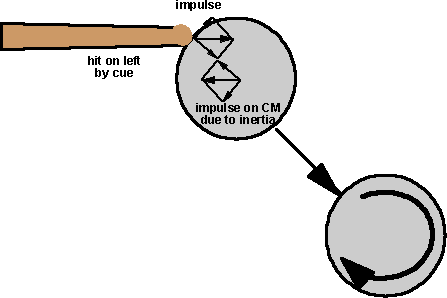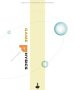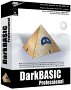# Table Top Physics - Snooker/pool - left side

A ball is hit by cue on the left, if we assume that the momentum is transferred only as an impulse, how do we work out the resulting linear and angular velocity of the ball?Assume the following:

• impulse = 1 kg m/s
• mass of ball = 1 kg
• radius of ball = r = 50 cm
• offset of cue = 2/5 r2 (chosen in hope of simplifying calculations, because this is the moment of inertia)

## Method 1

linear velocity = impulse / mass = 1 m/s in the direction of the impulse

angular velocity = offset * impulse / I = (2/5 r2)/(2/5 r2) = 1 rad/sec clockwise

## Method 2In this method the impulse is resolved into a component toward the centre of mass and a component at 90 degrees, then assume that the component toward the CM generates the linear velocity and the component at 90 degrees generates the angular velocity.

## Which is correct?

Can anyone help me here?

I think method 1 is correct because there is no justification for saying that the component toward the CM generates only the linear velocity and the component at 90 degrees generates only the angular velocity. However method 1 does not seem intuitively right, it seems that there should be some linear component to the right.

This is not a closed system so we cant use the conservation of angular momentum, is there a similar example which is a closed system, so that we can use conservation of angular momentum to check the result?

Where I can, I have put links to Amazon for books that are relevant to the subject, click on the appropriate country flag to get more details of the book or to buy it from them.Game Physics - This book has some useful stuff, its more of a textbook, not a step by step guide (although it does have a disc with a lot of C++ code). About the first third of the book is a physics textbook with theoretical exercises, the middle bit covers physics engine topics, and the last third of the book covers mathematical topics. I think I would use this book as a reference book to lookup the theory behind something I might be working on rather than a book to work through in order.

Commercial Software Shop

Where I can, I have put links to Amazon for commercial software, not directly related to the software project, but related to the subject being discussed, click on the appropriate country flag to get more details of the software or to buy it from them.Dark Basic Professional Edition - It is better to get this professional editionThis is a version of basic designed for building games, for example to rotate a cube you might do the following: make object cube 1,100 for x=1 to 360 rotate object 1,x,x,0 next x

This site may have errors. Don't use for critical systems.